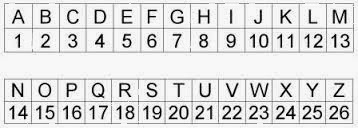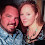## Saturday, January 11, 2014

### 33 Watch: The 13 Original Colonies & Undeniable Proof the United States is a Freemason CreationUse this chart to calculate Standard Method

﻿Use this chart to calculate Pythagorean Method

The Numerology of the First 13 Colonies, in order of statehood, from first to last:

DELAWARE 4+5+12+1+23+1+18+5 = 69 = 15 = 6
DELAWARE 4+5+3+1+5+1+9+5 = 33
• Delaware was established as the Nation's first state, December 7, 1787.  The numerology of this date is as follows: 12/7/1787 = 1+2+7+1+7+8+7 = 33
• Ask yourself, do you think the state equating to 33 in Gematria, and it being established on a date with a numerology of 33, is a coincidence?
• Remember, this Nation was founded by Masons, who have a highest degree of 33.
PENNSYLVANIA = 16+5+14+14+19+25+12+22+1+14+9+1 = 152 = 8
PENNSYLVANIA = 7+5+5+5+1+7+3+4+1+5+9+1 = 53 = 8 (Notice the 555 within)
• Pennsylvania was established December 12, 1787.  The numerology of this date is as follows:  12/12/1787 = 1+2+1+2+1+7+8+7 = 29 = 11
• The State's Gematria of 8, multiplied by the numerology of its founding date (11) equals 88, the Gematria of the name George Washington.  8 in numerology also symbolizes balance, strength and the infinite.
• There is also a 555 coded into the name, matching the height of the Washington Monument and the word "New", which is found in the third, ninth, eleventh and forty-seventh state.
• Pennsylvania is known as the "Keystone" State
• Keystone = 2+5+7+1+2+6+5+5 = 33
NEW JERSEY = 14+5+23 10+5+18+19+5+25 = 42+82 = 124 = 7
NEW JERSEY = 5+5+5 1+5+9+1+5+7 = 15+28 = 43 = 7
• New Jersey is one of four states with the word "new" in it.  New in Gematria = 555, the same height as the Washington Monument.  The state's with "new" in their name are New Jersey, New Hampsire, New York and New Mexico; the 3rd, 9th, 11th and 47th states respectively.  Do you see what is interesting about the numbers of those states?
• Hint (Also 47 = 11)
GEORGIA = 7+5+15+18+7+9+1 = 62 = 8
GEORGIA = 7+5+6+9+7+9+1 = 44 = 8
• Georgia is the fourth state and one that has a gematria of 44.  44 is associated with assassination, which might explain the bombings of the Atlanta Olympic Games on July 27, 1996.  The numerology of this date is as follows:  July 27 = 7/27 = 16 = 7.  1996 = 25 = 7.  Combined, we get 7/7.
• It might also be why hundreds of thousands of plastic coffins were discovered in that state, appearing ready for some sort of disaster.  Video here.
• Aleister Crowley wrote a book titled Magick:  Book 4.  The numerology of Magick = 44.  The book deals with Occult Numerology and Black Magic.  Black = 11 Magic = 33 (33+11 = 44)
• Georgia is also the home of the Georgia Guidestone, placed where they are March 22, 1980, with 10 commandments for a new age.  The first commandment is "Maintain the earth's population at 500 million."
CONNECTICUT = 3+15+14+14+5+3+20+9+3+21+20 = 127 = 10
CONNECTICUT = 3+6+5+5+5+3+2+9+3+3+2 = 46 = 10 (Notice the 555 within)
• Connecticut is the fifth state, and has the numbers 555 coded within it.  It is also the home of the political dynasty, The Bush's.
• More recently, it has become the home of the Sandy Hook Conspiracy and the movement against the Second Amendment.
MASSACHUSETTS = 13+1+19+19+1+3+8+21+19+5+20+20+19 = 168 = 15 = 6
MASSACHUSETTS = 4+1+1+1+1+3+8+3+1+5+2+2+1 = 33 = 6
• 11:11 is coded within the name Massachusetts
• Also, Massachusetts is equal to 33 in gematria
• 3+3 = 6, Massachusetts is the sixth state
MARYLAND = 13+1+18+25+12+1+14+4 = 88 = 16 = 7
MARYLAND = 4+1+9+7+3+1+5+4 = 34 = 7
• The name George Washington also equates to 88
• GEORGE = 39
• WASHINGTON = 49
• GEORGE WASHINGTON = 88 = MARYLAND
• 88 symbolizes the infinite, power and balance
SOUTH CAROLINA = 19+15+21+20+8 3+1+18+15+12+9+14+1 = 83+73 = 156 = 12 = 3
SOUTH CAROLINA = 1+6+3+2+8 3+1+9+6+3+9+5+1 = 20+37 = 57 = 12 = 3
NEW HAMPSHIRE = 14+5+23 8+1+13+16+19+8+9+18+5 = 42+97 = 139 = 13
NEW HAMPSHIRE = 5+5+5 8+1+4+7+1+8+9+9+5 = 15+52 = 67 = 13
• This is the second of four 555 aka new states.  It has a numerology that matches the number of stripes on the flag and original colonies.  It is also the ninth state.
• The state was founded June 21, 1788.  The numerology of this is 336+2+1+1+7+8+8 = 33
VIRGINIA = 22+9+18+7+9+14+9+1 = 89 = 17 = 8
VIRGINA = 4+9+9+7+9+5+9+1 = 53 = 8
• Virginia has the same numerology as Pennsylvania.  The original capital was in Philadelphia, Pennsylvania.  The modern Capital is in Virginia, in Washington D.C.  Perhaps a State with a numerology of 8 is important in this regard.
NEW YORK = 14+5+23 25+15+18+11 =42+69 = 111 = 3
NEW YORK = 5+5+5 7+6+9+2 = 15+24 = 39 = 12 = 3
• New York is the 11th State and third state to have 555 coded into it, following NJ and NH.
• It was founded July 26, 1788 = 7/26/1788 = 7+2+6+1+7+8+8 = 39
• York can also be reduced with the 'K' exception where the exception applies to K and V because they are the 11th and 22nd letters respectively.
• York = 7+6+9+11 = 33
• New Amsterdam, now New York City, was established February 2, the 33rd day of the year.
• Governor Clinton was the first Governor of New York
• Clinton = 3+3+9+5+2+6+5 = 33
NORTH CAROLINA = 14+15+18+20+8 3+1+18+15+12+9+14+1 = 75+73 = 148 = 13
NORTH CAROLINA = 5+6+9+2+8 3+1+9+6+3+9+5+1 = 30+37 = 67 = 13
• Stripes on the Flag
RHODE ISLAND = 18+8+15+4+5 9+19+12+1+14+4 = 50+59 = 109 = 10
RHODE ISLAND = 9+8+6+4+5 9+1+3+1+5+4 = 32+23 = 55 = 10
• The first 13 colonies begin with 33, and end with 33.  The next point is how.
• Rhode Island was founded May 29, 1790.  5/29/1790 = 5+2+9+1+7+9+0 = 33
• Also, 10 represents God and the divine.  If you add the numbers 1 through ten together, the sum = 55, which the numerology of is 10.  This is also equal to Rhode Island.
• 1+2+3+4+5+6+7+8+9+10 = 55 = 5+5 = 10 = Rhode Island
So there we have it, the first 13 colonies, as plotted by the Freemasons, and some of their numerological and Gematria significances.  Most interesting of all, is that Delaware is equal to 33, and was founded on a date that also equals to 33 in numerology.  It is also interesting to note the special numerological significances of the last of the 13 colonies; Rhode Island; and how it too was founded on a date with a numerology of 33.  The only other states to have this numerological significance are the sixth and ninth, Massachusetts which equates to 33 and New Hampshire which was founded on a date that equates to 33.

The next most significant curiosity, at least in my opinion, is that of the fourth state Georgia equates to 44 in Gematria.  Within the state of Georgia, you will find the Georgia Guidestones, which were placed there on March 22, 1980.  The first rule of the Georgia Guidestones is to "maintain the earth's population at 500 million."  As stated before, the number 44 is associated with assassination, and in order to get the world's population to 500 million, nearly 7 billion people would have to be assassinated, or 13 out of every 14 living people roughly.  In my original post on this blog, I talked about the next 9/11, and why I thought the Carolinas and Georgia might be a target.  This numerological revelation adds more plausibility to the theory.  That post can be read here.  Further, a movie titled. 4:44 The Last Day on Earth was released at the end of 2011, and reviewed in the New York Times on March 22, 2012, the same month and day the Georgia Guidestones were placed.

This is where it gets really interesting...
The 13 Colonies Combined:
Delaware = 6 (33)
Pennsylvania = 8
New Jersey = 7
Georgia = 8
Connecticut = 1
Massachusetts = 6 (33)
Maryland = 7
South Carolina = 3
New Hampshire = 4
Virginia = 8
New York = 3
North Carolina  = 4
Rhode Island = 1
Total = 66 = Woman = Associated with birth and creation, just like a new nation.
• Woman = 23+15+13+1+14 = 66
• Freedom = 6+18+5+5+4+15+13 = 66
• Thomas = 2+8+6+4+1+1 = 22 Jefferson = 1+5+6+6+5+9+1+6+5 = 44
• Thomas Jefferson = 22+44 = 66
• Thomas Jefferson wrote the Declaration of Independence, which was signed by 39
• (Pythagorean Method) Freedom = 6+9+5+5+4+6+4 = 39
• Route 66 was the nation's first highway  More here.
• The NFL began in 1966, or '66
• The Church of Satan was established in 1966
• There are 66 Books in the Bible
• 39 Old Testament
• 27 New Testament
• There are 39-floors at the United Nations in New York
• New York = 5+5+5+7+6+9+2 = 39
• York = 7+6+9+11 = 3
• Wicca = 23+9+3+3+1 = 39
• Pagan = 16+1+7+1+14 = 39
In other words, think about how carefully planned out the names of these 13 colonies are.  These numbers equating to what they do are not an accident or coincidence.

Three of the thirteen colonies were established on dates with 33 numerology, much like three of the fifty states have 33 coded into their names.
• Delaware = 12/7/1787 = 1+2+7+1+7+8+7 = 33
• New Hampshire = 6/21/1788 = 6+2+1+1+7+8+8 = 33
• Rhode Island = 5/29/1790 = 5+2+9+1+7+9+0 = 33
• Notice Delaware is the first, NH the ninth and Rhode Island the thirteenth and last
Then there are the fifty states.
• Delaware = 4+5+3+1+5+1+9+5 = 33
• Massachusetts = 4+1+1+1+1+3+8+3+1+5+2+2+1 = 33
• Hawaii = 8+1+5+1+9+9 = 33
• Notice the first, sixth and fiftieth and final state, much like the 13-Colonies
If you find any other curiosities relating to this post and pertaining to the 13 original colonies, please don't hesitate to share.  Thank you for reading.

#### 1 comment:

1.How I Got My Ex Husband Back...........

I am Shannon by name. Greetings to every one that is reading this testimony. I have been rejected by my husband after three(3) years of marriage just because another woman had a spell on him and he left me and the kid to suffer. one day when i was reading through the web, i saw a post on how this spell caster on this address Makospelltemple@yahoo.com , have help a woman to get back her husband and i gave him a reply to his address and he told me that a woman had a spell on my husband and he told me that he will help me and after 2 days that i will have my husband back. i believed him and today i am glad to let you all know that this spell caster have the power to bring lovers back. because i am now happy with my husband. Thanks for Dr.Mako. His email: Makospelltemple@yahoo.com OR. his phone number: +2348108737816.